24 Matching Annotations
1. Mar 2020
2. intellspot.com intellspot.com
1. In the interval scale, there is no true zero point or fixed beginning. They do not have a true zero even if one of the values carry the name “zero.” For example, in the temperature, there is no point where the temperature can be zero. Zero degrees F does not mean the complete absence of temperature. Since the interval scale has no true zero point, you cannot calculate Ratios. For example, there is no any sense the ratio of 90 to 30 degrees F to be the same as the ratio of 60 to 20 degrees. A temperature of 20 degrees is not twice as warm as one of 10 degrees.

Interval data:

• show not only order and direction, but also the exact differences between the values
• the distances between each value on the interval scale are meaningful and equal
• no true zero point
• no fixed beginning
• no possibility to calculate ratios (only add and substract)
• e.g.: temperature in Fahrenheit or Celsius (but not Kelvin) or IQ test
2. As the interval scales, Ratio scales show us the order and the exact value between the units. However, in contrast with interval scales, Ratio ones have an absolute zero that allows us to perform a huge range of descriptive statistics and inferential statistics. The ratio scales possess a clear definition of zero. Any types of values that can be measured from absolute zero can be measured with a ratio scale. The most popular examples of ratio variables are height and weight. In addition, one individual can be twice as tall as another individual.

Ratio data is like interval data, but with:

• absolute zero
• possibility to calculate ratio (e.g. someone can be twice as tall)
• possibility to not only add and subtract, but multiply and divide values
• e.g.: weight, height, Kelvin scale (50K is 2x hot as 25K)

#### URL

3. towardsdatascience.com towardsdatascience.com
1. If you are interested in exploring the dataset used in this article, it can be used straight from S3 with Vaex. See the full Jupyter notebook to find out how to do this.

Example of EDA in Vaex ---> Jupyter Notebook

2. Vaex is an open-source DataFrame library which enables the visualisation, exploration, analysis and even machine learning on tabular datasets that are as large as your hard-drive. To do this, Vaex employs concepts such as memory mapping, efficient out-of-core algorithms and lazy evaluations.

Vaex - library to manage as large datasets as your HDD, thanks to:

• memory mapping
• efficient out-of-core algorithms
• lazy evaluations.

All wrapped in a Pandas-like API

3. The first step is to convert the data into a memory mappable file format, such as Apache Arrow, Apache Parquet, or HDF5

Before opening data with Vaex, we need to convert it into a memory mappable file format (e.g. Apache Arrow, Apache Parquet or HDF5). This way, 100 GB data can be load in Vaex in 0.052 seconds!

Example of converting CSV ---> HDF5.

4. The describe method nicely illustrates the power and efficiency of Vaex: all of these statistics were computed in under 3 minutes on my MacBook Pro (15", 2018, 2.6GHz Intel Core i7, 32GB RAM). Other libraries or methods would require either distributed computing or a cloud instance with over 100GB to preform the same computations.

Possibilities of Vaex

5. AWS offers instances with Terabytes of RAM. In this case you still have to manage cloud data buckets, wait for data transfer from bucket to instance every time the instance starts, handle compliance issues that come with putting data on the cloud, and deal with all the inconvenience that come with working on a remote machine. Not to mention the costs, which although start low, tend to pile up as time goes on.

AWS as a solution to analyse data too big for RAM (like 30-50 GB range). In this case, it's still uncomfortable:

• managing cloud data buckets
• waiting for data transfer from bucket to instance every time the instance starts
• handling compliance issues coming by putting data on the cloud
• dealing with remote machines
• costs

#### URL

4. towardsdatascience.com towardsdatascience.com
1. when AUC is 0.5, it means model has no class separation capacity whatsoever.

If AUC = 0.5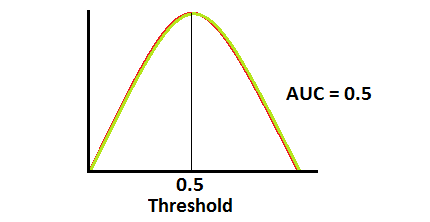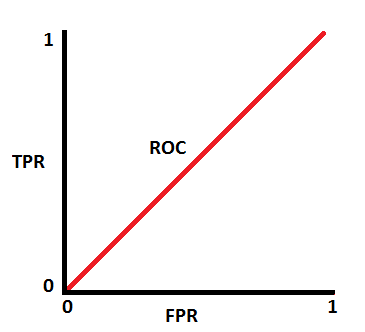2. ROC is a probability curve and AUC represents degree or measure of separability. It tells how much model is capable of distinguishing between classes.

ROC & AUC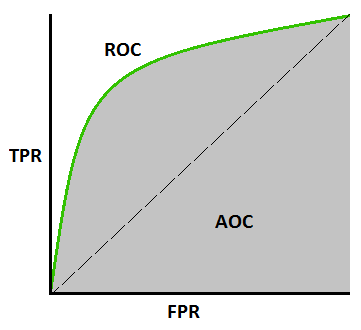3. In multi-class model, we can plot N number of AUC ROC Curves for N number classes using One vs ALL methodology. So for Example, If you have three classes named X, Y and Z, you will have one ROC for X classified against Y and Z, another ROC for Y classified against X and Z, and a third one of Z classified against Y and X.

Using AUC ROC curve for multi-class model

4. When AUC is approximately 0, model is actually reciprocating the classes. It means, model is predicting negative class as a positive class and vice versa

If AUC = 0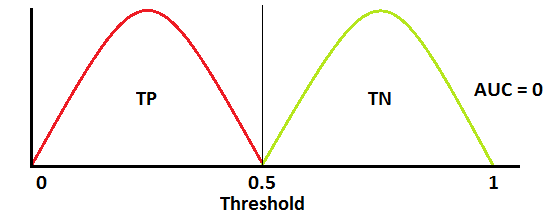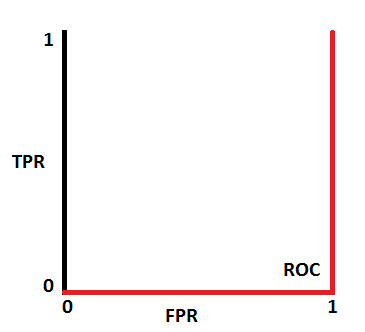5. AUC near to the 1 which means it has good measure of separability.

If AUC = 1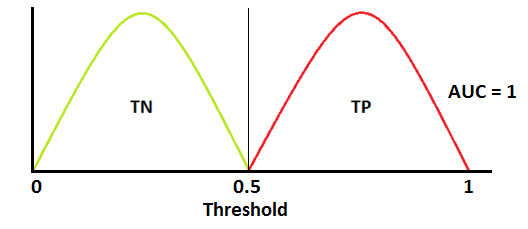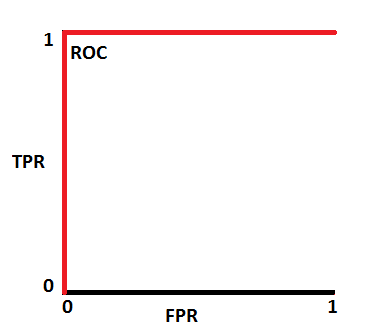#### URL

5. p.migdal.pl p.migdal.pl
1. Creating meticulous tests before exploring the data is a big mistake, and will result in a well-crafted garbage-in, garbage-out pipeline. We need an environment flexible enough to encourage experiments, especially in the initial place.

Overzealous nature of TDD may discourage from explorable data science

#### URL

1. LR is nothing but the binomial regression with logit link (or probit), one of the numerous GLM cases. As a regression - itself it doesn't classify anything, it models the conditional (to linear predictor) expected value of the Bernoulli/binomially distributed DV.

Linear Regression - the ultimate definition (it's not a classification algorithm!)

It's used for classification when we specify a 50% threshold.

#### URL

7. victorzhou.com victorzhou.com
1. BOW is often used for Natural Language Processing (NLP) tasks like Text Classification. Its strengths lie in its simplicity: it’s inexpensive to compute, and sometimes simpler is better when positioning or contextual info aren’t relevant

Usefulness of BOW:

• simplicity
• low on computing requirements
2. Notice that we lose contextual information, e.g. where in the document the word appeared, when we use BOW. It’s like a literal bag-of-words: it only tells you what words occur in the document, not where they occurred

The analogy behind using bag term in the bag-of-words (BOW) model.

3. Here’s my preferred way of doing it, which uses Keras’s Tokenizer class

Keras's Tokenizer Class - Victor's preferred way of implementing BOW in Python

#### URL

8. martinfowler.com martinfowler.com
1. process that takes input data through a series of transformation stages, producing data as output

Data pipeline

#### URL

9. victorzhou.com victorzhou.com
1. Softmax turns arbitrary real values into probabilities

Softmax function -

• outputs of the function are in range [0,1] and add up to 1. Hence, they form a probability distribution
• the calcualtion invloves e (mathematical constant) and performs operation on n numbers: $$s(x_i) = \frac{e^{xi}}{\sum{j=1}^n e^{x_j}}$$
• the bigger the value, the higher its probability
• lets us answer classification questions with probabilities, which are more useful than simpler answers (e.g. binary yes/no)

#### URL

1. 1. Logistic regression IS a binomial regression (with logit link), a special case of the Generalized Linear Model. It doesn't classify anything *unless a threshold for the probability is set*. Classification is just its application. 2. Stepwise regression is by no means a regression. It's a (flawed) method of variable selection. 3. OLS is a method of estimation (among others: GLS, TLS, (RE)ML, PQL, etc.), NOT a regression. 4. Ridge, LASSO - it's a method of regularization, NOT a regression. 5. There are tens of models for the regression analysis. You mention mainly linear and logistic - it's just the GLM! Learn the others too (link in a comment). STOP with the "17 types of regression every DS should know". BTW, there're 270+ statistical tests. Not just t, chi2 & Wilcoxon

5 clarifications to common misconceptions shared over data science cheatsheets on LinkedIn

#### URL

11. news.ycombinator.com news.ycombinator.com
1. 400 sized probability sample (a small random sample from the whole population) is often better than a millions sized administrative sample (of the kind you can download from gov sites). The reason is that an arbitrary sample (as opposed to a random one) is very likely to be biased, and, if large enough, a confidence interval (which actually doesn't really make sense except for probability samples) will be so narrow that, because of the bias, it will actually rarely, if ever, include the true value we are trying to estimate. On the other hand, the small, random sample will be very likely to include the true value in its (wider) confidence interval

#### URL

1. An exploratory plot is all about you getting to know the data. An explanatory graphic, on the other hand, is about telling a story using that data to a specific audience.

Exploratory vs Explanatory plot

#### URL

13. Dec 2018
14. www.tandfonline.com www.tandfonline.com
1. ggplot2 and dplyr are clear intellectual contributions because they provide tools (grammars) for visualization and data manipulation, respectively. The tools make the tasks radically easier by providing clear organizing principles coupled with effective code. Users often remark on the ease of manipulating data with dplyr and it is natural to wonder if perhaps the task itself is trivial. We claim it is not. Many probability challenges become dramatically easier, once you strike upon the “right” notation. In both cases, what feels like a matter of notation or syntax is really a more about exploiting the “right” abstraction.

This is a fascinating example to me of both an important intellectual contribution that is embodied in the code itself, and also how extremely important work can be invisible or taken for granted because of how seamlessly or easily it becomes integrated into other research,